# Explanations for solar physics computing page

This document explains values, tables and other matters on solar physics computing pages.

## Equation of state and opacity tables

Computing solar internal structure and evolution relies heavily on plasma physics, including equation of state and opacity. Since equation of state and opacity are not easy to calculate they are usually used in the form of tables which are interpolated. OPAL opacity and EOS tables can be obtained from Livermore Lavoratories web page.

### Equation of state tables

is040423.etf: SAHA-S tables, interpolation values: T, rho, X, Z. Gryaznov V.K., Ayukov S.V., Baturin V.A., Iosilevskiy I.L., Starostin A.N., Fortov V.E.: In: Equation-of-State and Phase-Transition Issues in Models of Ordinary Astrophysical Matter, Eds. V.Celebonovic, W.Dappen, D.Gough. AIP Conference Proceedings, 731, New York, 147 (2004)

is040423.ete: SAHA-S tables, interpolation values: T, rho, X. Z is fixed at 0.02. See reference above.

opal2005.etf: OPAL EOS, interpolation values: T, rho, X, Z. Downloaded from OPAL site, marked there as "2005", seems to be unpublished.

opal2001.etf: OPAL EOS, interpolation values: T, rho, X, Z. Rogers F.J., Nayfonov A. Astrophys. J., 576, 1064 (2002).

opal1996.etf: OPAL EOS, interpolation values: T, rho, X, Z. Rogers, F.J., Swenson, F.J., Iglesias, C.A.: Astrophys. J., 456, 902 (1996)

mhd5.ete: MHD equation of state, interpolation values: T, rho, X. Z is fixed at 0.02. Provided by W.Dappen. References are: Hummer, D.G., and Mihalas, D. 1988, Astrophys. J., 331, 794; Mihalas, D., Dappen W., and Hummer, D.G. 1988, Astrophys. J., 331, 815; Dappen, W., Mihalas, D., Hummer, D.G., and Mihalas, B.W. 1988, Astrophys. J., 332, 261.

### Opacity tables

opal92: Rogers, F.J., Iglesias, C.A.: Astrophys. J. Suppl. Ser. 79, 507 (1992)
opal95: Iglesias, C.A., Rogers, F.J.: Astrophys. J., 464, 943 (1996)
ferguson2005: Ferguson, J.W., Alexander, D.R., Allard, F., Barman, T., Bodmarik, J.G., Hauscildt, P.H., Heffner-Wong, A., Tamanai, A.: Astrophys. J. 623, 585 (2005)

## Mixture

`Mixture' is a set of relative abundances of chemical elements. Since H and He cannot be reliably determined from observations the term mixture in solar physics is related to elements heavier than helium. The chemical composition is a part of input data for opacities and equation of state; it can be either pair of X and Z; X, ZCNO, ZNe, ZFeSi; X and 19 individual Z components (see Input data below). When heavy element contents is specified as single Z value or ZC/ZN/ZF combo the mixture is used to compute individual element abundances. However not all EOS/opacity tables take into account individual element abundances. Right now only opal_f2005.otg table uses 19 heavy element abundances as input (this may change in the future). All other tables ignore mixture and use only total Z as input. Note that every table assumes some mixture internally but this mixture cannot be changed. Again if one specifies all 19 Zi for opal_f2005.otg table then mixture is also not used. So mixture is only used for opal_f2005.otg opacity when one Z or three groups ZCNO, ZNe, ZFeSi are specified.

The following mixtures are available: AG89, GN93, GS98, AGS05, LPG09, AGSS09.

## Output values

The following values can be calculated:

"Symbol" is what appears in the header of the results table.
Value Symbol Formulae Unit, notes
Pressure P dyn/cm^2; includes radiative pressure
Reduced pressure PISpecific internal energy U erg/g
Logarithmic partial derivative hi_tdimensionless
Logarithmic partial derivative hi_rho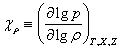dimensionless
Logarithmic partial derivative hi_x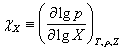dimensionless
Expansion coefficient Q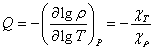dimensionless
Specific heat at constant volume Cv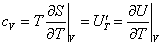erg/(g*K)
Specific heat at constant pressure Cperg/(g*K)
Logarithmic partial derivative ksi_rho dlogU/dlogRHO dimensionless
Adiabatic exponent Gamma3 G3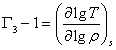dimensionless
Adiabatic temperature gradient nabladimensionless
Adiabatic exponent Gamma1 G1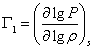dimensionless
Sound speed cs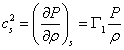cm/sec
Rosseland opacity K cm^2/g
Nuclear energy generation rate eps erg/(gram*sec); PP-chain and CNO cycle. He3 is in equilibrium
Hydrogen burning rate dXdt 1/sec

## Input data

The following values must be input: temperature T (in Kelvin degrees), density rho (g/cm3), hydrogen mass fraction X and heavy element mass fraction Z. Helium mass fraction is assumed to be equal to 1-X-Z.

Formal limits for lgT are 3.0...9.0, for lgQ -- (Q=rho/(T/1e6)^3) -8.0...2.0, for X -- 0.0...0.9, for Z -- 0.0...0.025. However most tables are not rectangular, and data is missing in some points (T, rho, X, Z). When data is missing, execution stops and nothing is printed.

Heavy elements can be also specified as three components (CNO, Ne, FeSi) or 19 components (C, N, O, Ne, Na, Mg, Al, Si, P, S, Cl, Ar, K, Ca, Ti, Cr, Mn, Fe, Ni). These formats are not very useful with current EOS/opacity tables as most tables cannot be interpolated by individual element abundances. See Tables above on tables interpolation capabilities.